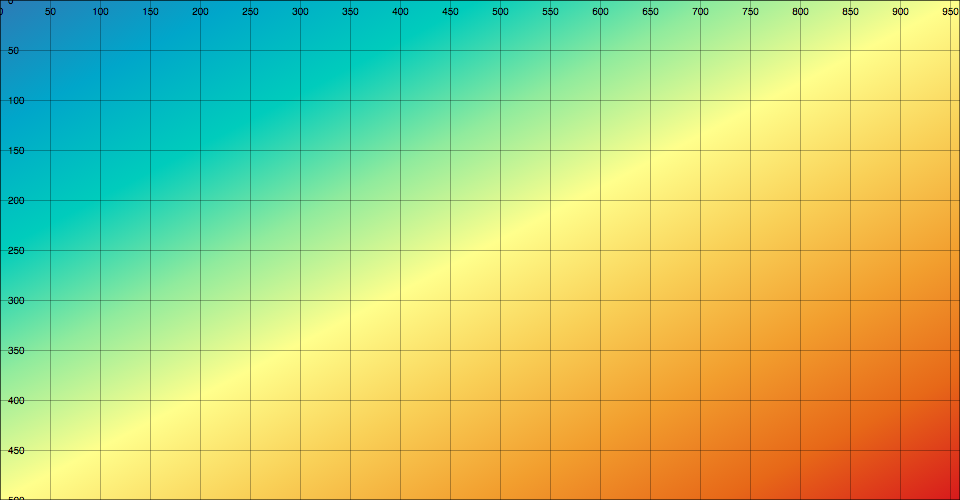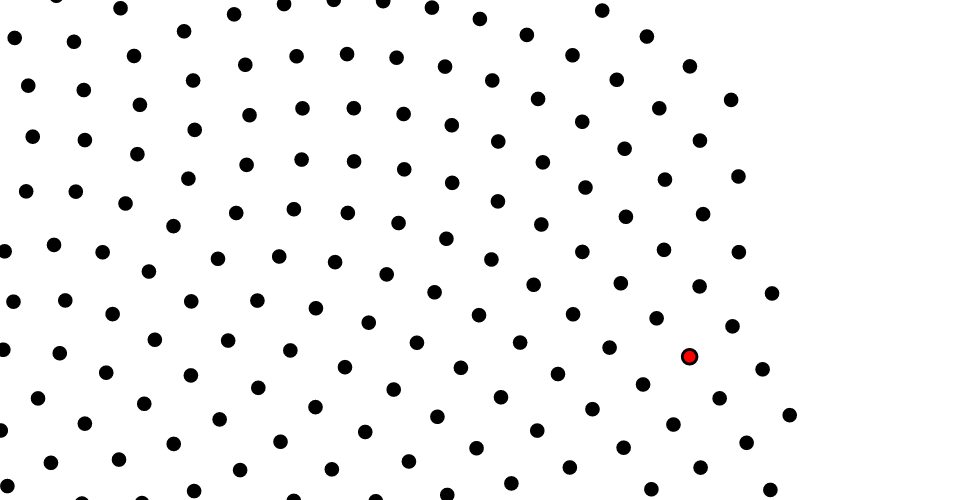# # d3-zoom## # Installing

`NPM` 安装: `npm install d3-zoom`. 此外还可以下载 latest release. 可以直接从 d3js.org, 以 standalone library 或作为 D3 4.0 的一部分引入. 支持 `AMD`, `CommonJS` 以及基本的标签引入形式，如果使用标签引入则会暴露全局 `d3` 变量:

``````<script src="https://d3js.org/d3-color.v1.min.js"></script>
<script src="https://d3js.org/d3-dispatch.v1.min.js"></script>
<script src="https://d3js.org/d3-ease.v1.min.js"></script>
<script src="https://d3js.org/d3-interpolate.v1.min.js"></script>
<script src="https://d3js.org/d3-selection.v1.min.js"></script>
<script src="https://d3js.org/d3-timer.v1.min.js"></script>
<script src="https://d3js.org/d3-transition.v1.min.js"></script>
<script src="https://d3js.org/d3-drag.v1.min.js"></script>
<script src="https://d3js.org/d3-zoom.v1.min.js"></script>
<script>

var zoom = d3.zoom();

</script>
``````

## # API Reference

Event Listening Element Zoom Event Default Prevented?
mousedown⁵ selection start no¹
mousemove² window¹ zoom yes
mouseup² window¹ end yes
dragstart² window - yes
selectstart² window - yes
click³ window - yes
dblclick selection multiple yes
wheel⁸ selection zoom⁷ yes
touchstart selection multiple no⁴
touchmove selection zoom yes
touchend selection end no⁴
touchcancel selection end no⁴

¹ 在 `iframe` 之外捕获事件的必要条件; 参考 d3-drag#9.
² 只适用于以鼠标为基础的活动; 参考 d3-drag#9.
³ 只在一些基于鼠标的手势之后立即应用; 参考 zoom.clickDistance.
⁴ 在触摸输入上允许 click emulation(点击模拟) 的必要条件; 参考 d3-drag#9.
⁵ 忽略触摸事件结束后的 `500ms` 之内的事件; 假设 click emulation(点击模拟).
⁶ 双击和双击启动一个转换，它会发出开始、缩放和结束事件。
⁷ 第一次滚轮事件发出一个启动事件; 当在 `150ms` 之内没有再次收到滚轮事件时，就会发出一个结束事件.
⁸ 如果已经达到了 scale extent 的限制，则忽略.

# d3.zoom() <源码>

# zoom(selection) <源码>

``````selection.call(d3.zoom().on("zoom", zoomed));
``````

``````selection.on(".zoom", null);
``````

``````selection
.call(zoom)
.on("wheel.zoom", null);
``````

# zoom.transform(selection, transform) <源码>

``````selection.call(zoom.transform, d3.zoomIdentity);
``````

``````selection.transition().duration(750).call(zoom.transform, d3.zoomIdentity);
``````

# zoom.translateBy(selection, x, y) <源码>

# zoom.translateTo(selection, x, y) <源码>

# zoom.scaleBy(selection, k) <源码>

# zoom.scaleTo(selection, k) <源码>

# zoom.constrain([constrain]) <源码>

``````function constrain(transform, extent, translateExtent) {
var dx0 = transform.invertX(extent) - translateExtent,
dx1 = transform.invertX(extent) - translateExtent,
dy0 = transform.invertY(extent) - translateExtent,
dy1 = transform.invertY(extent) - translateExtent;
return transform.translate(
dx1 > dx0 ? (dx0 + dx1) / 2 : Math.min(0, dx0) || Math.max(0, dx1),
dy1 > dy0 ? (dy0 + dy1) / 2 : Math.min(0, dy0) || Math.max(0, dy1)
);
}
``````

# zoom.filter([filter]) <源码>

``````function filter() {
return !d3.event.button;
}
``````

# zoom.touchable([touchable]) <源码>

``````function touchable() {
return "ontouchstart" in this;
}
``````

# zoom.wheelDelta([delta]) <源码>

``````function wheelDelta() {
return -d3.event.deltaY * (d3.event.deltaMode ? 120 : 1) / 500;
}
``````

`delata` 函数返回的值 Δ 定义了 WheelEvent 与缩放量的影响。缩放因子 transform.k 会乘以 2Δ。例如 Δ + 1 会使缩放因子增加一倍而 Δ - 1 会使缩放因子减少一倍。

# zoom.extent([extent]) <源码>

# zoom.scaleExtent([extent]) <源码>

``````selection
.call(zoom)
.on("wheel", function() { d3.event.preventDefault(); });
``````

# zoom.translateExtent([extent]) <源码>

# zoom.clickDistance([distance]) <源码>

# zoom.duration([duration]) <源码>

``````selection
.call(zoom)
.on("dblclick.zoom", null);
``````

# zoom.interpolate([interpolate]) <源码>

# zoom.on(typenames[, listener]) <源码>

typenames 是一个包含一个或多个由空格隔开的 typename 的字符串。每个 typename 都是一个 type，后面可以跟一个由 `.` 隔开的 name, 比如 `zoom.foo``zoom.bar`；通过 `name` 可以为同一种 type 同时指定多个事件监听器，type 为以下几种:

• `start` - `zooming` 开始之后(比如`mousedown`)
• `zoom` - `zoom` 的变换发生了变化(比如 `mousemove`)
• `end` - `zoom` 结束(比如 `mouseup`)

## # Zoom Events

zoom event listener 被调用时，d3.event 被设置为当前缩放事件。event 对象暴露以下几个字段:

• event.target - 关联的 zoom behavior.
• event.type - 字符串 “start”, “zoom” 或 “end”; 参考 zoom.on.
• event.transform - 当前 zoom transform.
• event.sourceEvent - 底层输入事件，比如 `mousemove``touchmove`.

## # Zoom Transforms

# d3.zoomTransform(node) <源码>

``````var transform = d3.zoomTransform(selection.node());
``````

event listener 的上下文中，node 通常是接收事件输入的元素(应该等价于 event.transform)，this:

``````var transform = d3.zoomTransform(this);
``````

k 0 tx
0 k ty
0 0 1

(这个矩阵只能表示缩放和平移，未来可能会支持旋转，尽管不会向后兼容)。坐标 ⟨x,y⟩ 被变换到 ⟨xk + tx,yk + ty⟩。变换对象暴露以下属性:

• transform.x - 在x-轴上的平移量 tx
• transform.y - 在y-轴上的平移量 ty
• transform.k - 缩放因子 k

``````var t = d3.zoomIdentity.translate(x, y).scale(k);
``````

``````context.translate(transform.x, transform.y);
context.scale(transform.k, transform.k);
``````

``````div.style("transform", "translate(" + transform.x + "px," + transform.y + "px) scale(" + transform.k + ")");
div.style("transform-origin", "0 0");
``````

``````g.attr("transform", "translate(" + transform.x + "," + transform.y + ") scale(" + transform.k + ")");
``````

``````g.attr("transform", transform);
``````

# transform.scale(k) <源码>

# transform.translate(x, y) <源码>

# transform.apply(point) <源码>

# transform.applyX(x) <源码>

# transform.applyY(y) <源码>

# transform.invert(point) <源码>

# transform.invertX(x) <源码>

# transform.invertY(y) <源码>

# transform.rescaleX(x) <源码>

``````function rescaleX(x) {
var range = x.range().map(transform.invertX, transform),
domain = range.map(x.invert, x);
return x.copy().domain(domain);
}
``````

# transform.rescaleY(y) <源码>

``````function rescaleY(y) {
var range = y.range().map(transform.invertY, transform),
domain = range.map(y.invert, y);
return y.copy().domain(domain);
}
``````

# transform.toString() <源码>

``````function toString() {
return "translate(" + this.x + "," + this.y + ") scale(" + this.k + ")";
}
``````

# d3.zoomIdentity <源码>